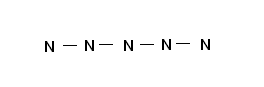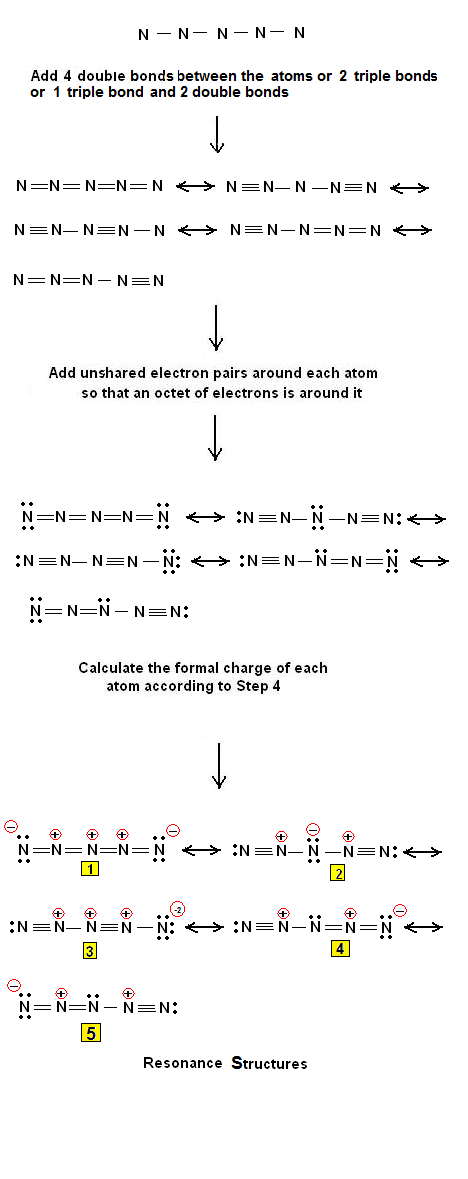Method of drawing the Lewis Structures of N5+ - #29 | Chemistry Net

# Method of drawing the Lewis Structures of N5+ - #29

A simple procedure for writing Lewis structures is given in a previous article entitled “Lewis Structures and the Octet Rule”. Relevant worked examples were given in the following articles: Examples #1, #2, #3 , #4, #5, #6,  #7#8, #9, #10, #11, #12, #13, #14 #15, #16, #17, #18, #19, #20, #21, #22,  #23,  #24, #25, #26, #27 and #28.

Let us consider the case of  the pentazenium or pentanitrogen N5+ cation. This highly explosive cation was first synthesized and isolated as a salt by K.O. Christie in 1999 by the reaction of  N2F+     with HN3 . The N5+ cation was the first new all nitrogen species to be synthesized in isolable quantities in more than a century, and only the third ever produced. Prior to this nitrogen cation only molecular N2 , isolated in 1772 and the azide ion N3- , first synthesized in 1890, had ever been isolated. The overall objective of Christie's research was the synthesis and characterization of polynitrogen, high-nitrogen and high-oxy gen energetic materials, useful as propellants or explosive ingredients.

Step 1: Connect the atoms with single bonds.Fig. 1: Connecting the atoms of  the  N5+ cation with single bonds

Step 2: Calculate the # of electrons in π bonds (multiple bonds) using  formula (1)

Where n in this case is 5 since N5+ consists of 5 atoms.
Where V = (5 * 5 ) - charge  = 25 – 1 = 24, V is the number of the valence electrons in the ion.
Therefore, P = 6n + 2 – V = 6 *5 + 2 – 24 = 8   \  there are: 1) either 4 double bonds   2)  or 2 triple bonds   3) or 1 triple and 2 double bonds.

Step 3 & 4: The Lewis structure of N5+  is as follows:Figure 2:  Lewis electron dot structures for the N5+ ion.  The least plausible structures are #1 (+ charges adjacent to each other) and #3 (large charge separation).

Therefore, five resonance electron dot structures were derived for the N5+ion.